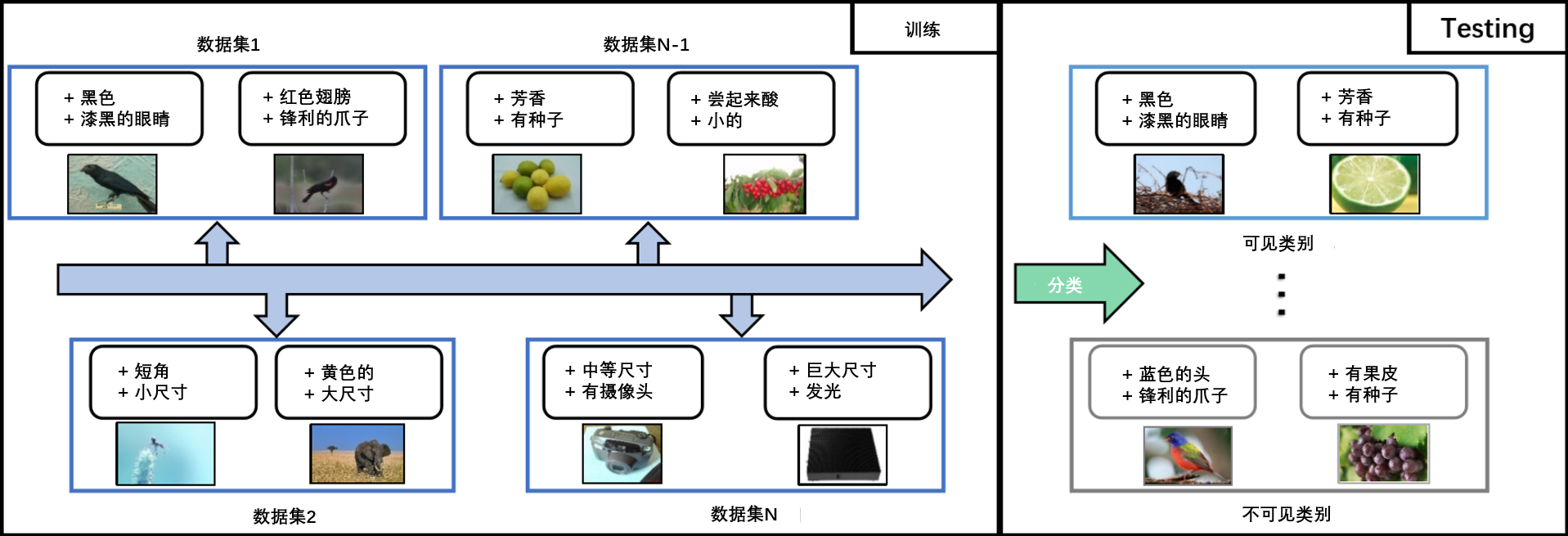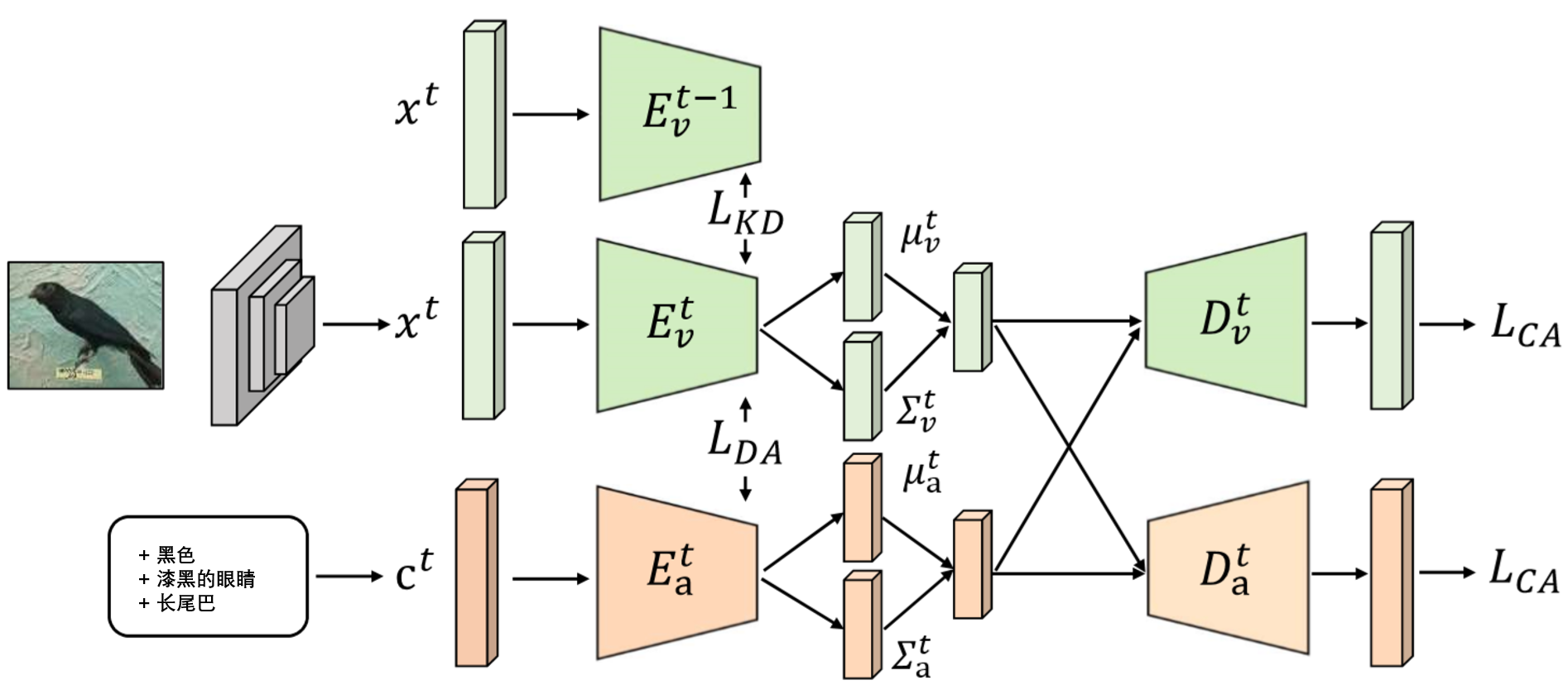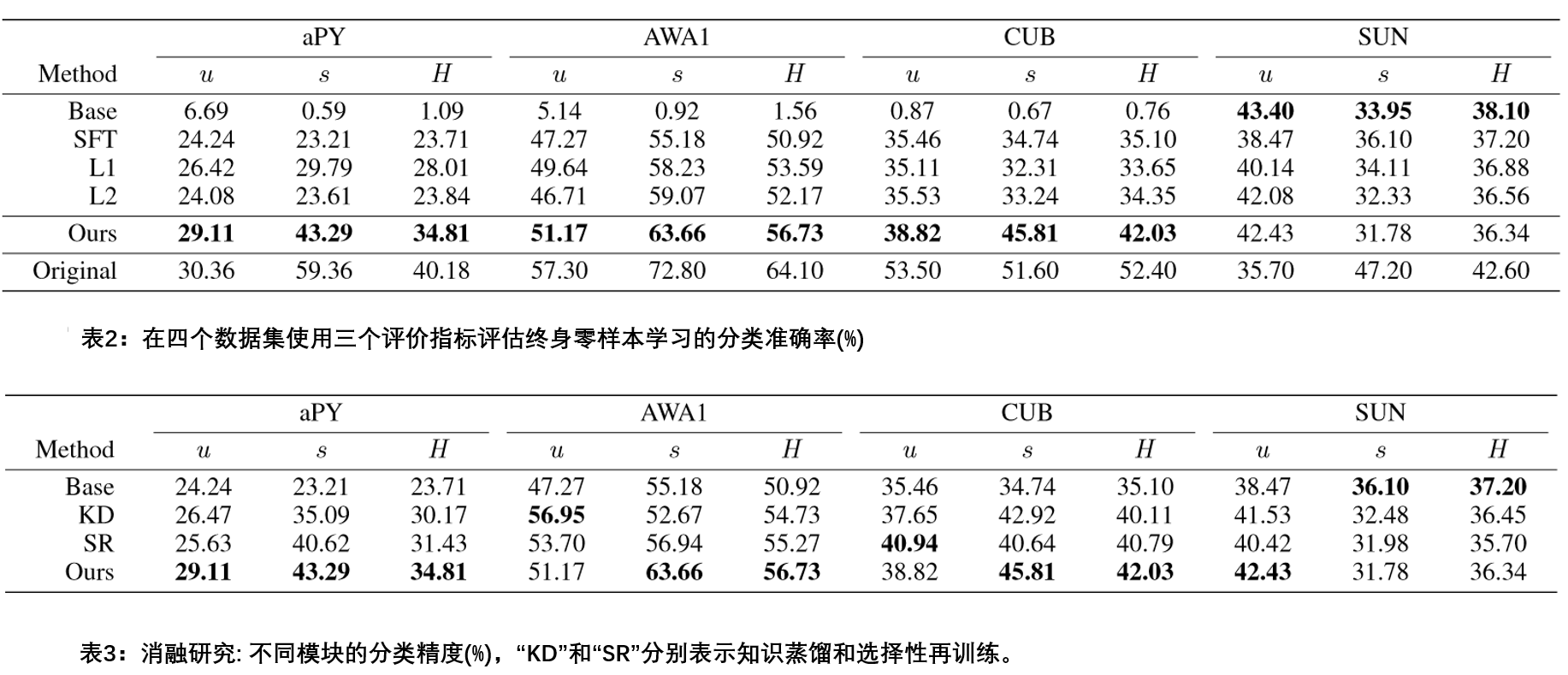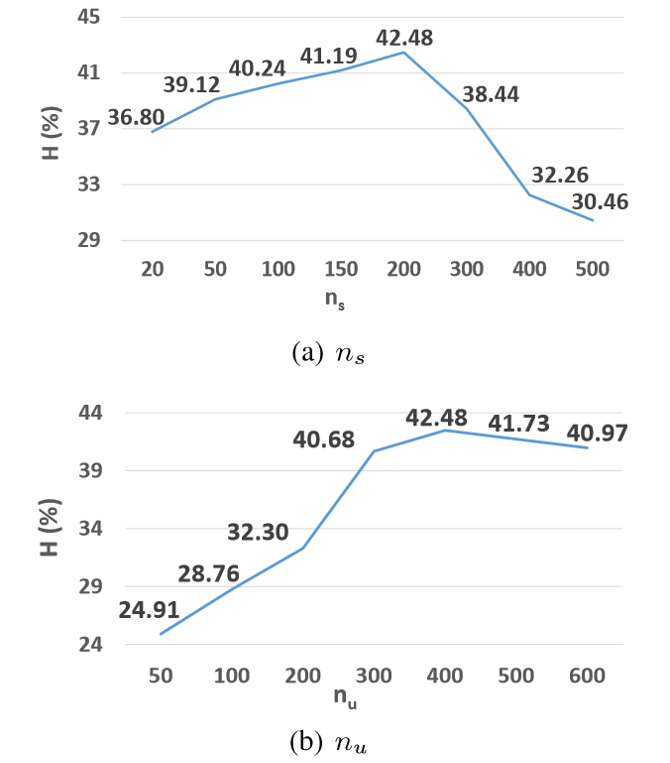# Lifelong Zero-Shot Learning(论文翻译)

https://www.ijcai.org/Proceedings/2020/0077.pdf

## 1. 介绍

• 据我们所知，我们是第一个提出并解决终身零样本学习问题的。我们以一种新颖的方式设计了终身零样本学习的基准和评估协议。

• 针对不同数据集的异构语义嵌入的挑战，我们采用了可以固定相应任务的潜在空间的VAEs算法去获得统一的语义嵌入。

• 利用选择性再训练提高不同数据集的分类空间之间的相似性，并通过知识蒸馏损失来监督，规范了知识从之前的任务向当前任务转移的过程。

• 在提出的基准上的大量的实验结果证明了我们提出的方法的有效性，它显著优于最先进的零样本学习方法。## 2. 相关工作

### 2.2 终身学习## 3. 方法

### 3.4 选择性再训练

1: 冻结参数$W^{t-1}_L$，$S^t={o_t}$
2: 微调网络
3: $\text{for l = L,…,l do}$
4: 添加神经元$i$到$S^t$，如果存在一些神经元$j \in S$，且$W_{l,ij}^{t-1}≠0$
5: $\text{end for}$
6: 微调选择的参数$W^t_S$

APY64153392012
AWA185304754010
CUB3121178815050
SUN1021434064572

## 4. 实验

### 4.1 基准和评估标准

• u：是对每类带有预测标签集的不可见类别的测试图像进行分类的平均准确率，用于衡量识别不可见类的能力。

• s：是对每类带有预测标签集的可见类的测试图像进行分类的平均准确率，用于衡量识别增量可见类的能力。

• H：u和s的调和均值，公式为：$H=\frac{2×u×s}{u+s}$。

### 4.3 与现存基准程序的比较

(a) 顺序微调(SFT): 当一个新任务按顺序到达时，模型被微调，该模型的参数从在前一个任务训练或微调的模型进行初始化。
(b) L2正则化(L2): 在每个任务t上，$Wt$初始化为$W{t−1}$，在$Wt$和$W{t−1}$之间持续进行L2正则化训练。
(C) L1正则化(L1): 在每个任务t上，$Wt$初始化为$W{t−1}$，在$Wt$和$W{t−1}$之间持续进行L1正则化训练。### 4.4 消融研究